Courses

# NCERT Solutions - Chapter 12: Exponents and Powers, Maths, Class 8 | EduRev Notes

## Mathematics (Maths) Class 8

Created by: Indu Gupta

## Class 8 : NCERT Solutions - Chapter 12: Exponents and Powers, Maths, Class 8 | EduRev Notes

The document NCERT Solutions - Chapter 12: Exponents and Powers, Maths, Class 8 | EduRev Notes is a part of the Class 8 Course Mathematics (Maths) Class 8.
All you need of Class 8 at this link: Class 8

Exercise 12.1

Question 1:

Evaluate:

(i) 3-2

(ii) (-4)-2

(iii) (1/2)-5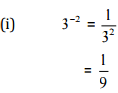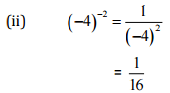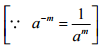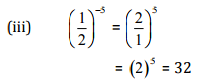Question 2:

Simplify and express the result in power notation with positive exponent: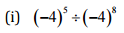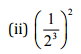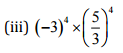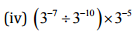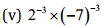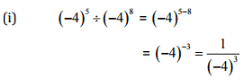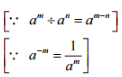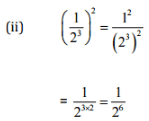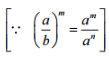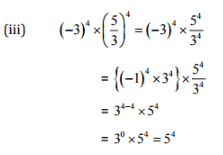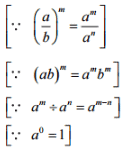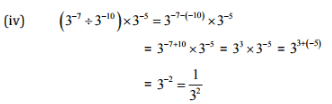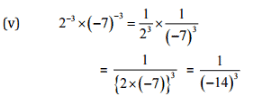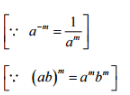Question 3:

Find the value of: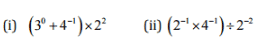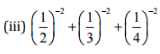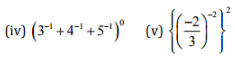(i)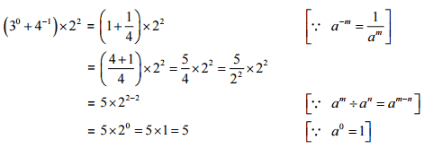(ii)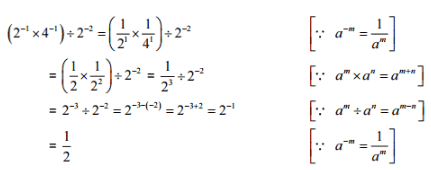(iii)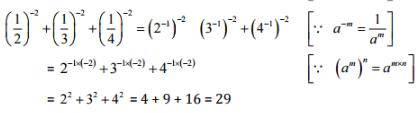(iv)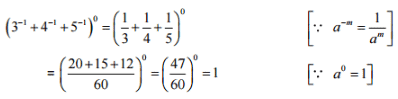(v)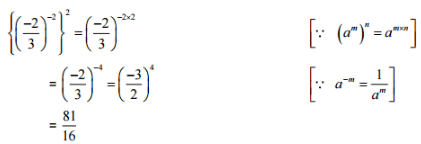Question 4:

Evaluate: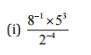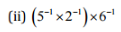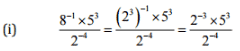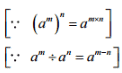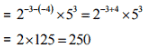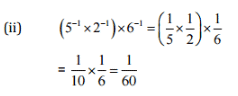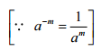Question 5: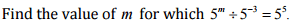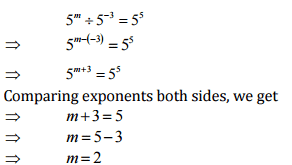Question 6:

Evaluate: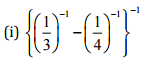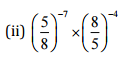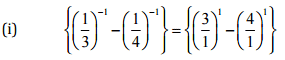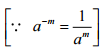= (3-4) = -1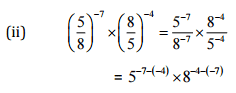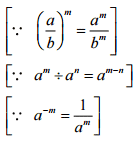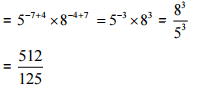Question 7:

Simplify: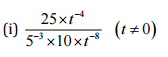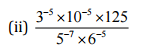(i)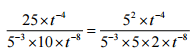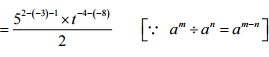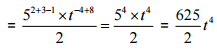(ii)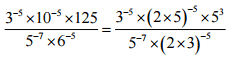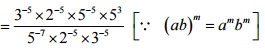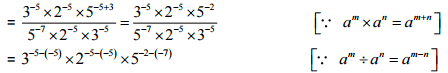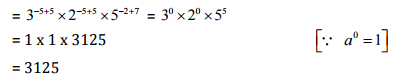Exercise 12.2

Question 1:

Express the following numbers in standard form:

(i) 0.0000000000085

(ii) 0.00000000000942

(iii) 6020000000000000

(iv) 0.00000000837

(v) 31860000000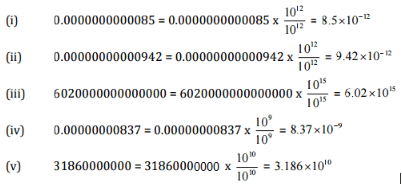Question 2:

Express the following numbers in usual form:

[i] 3.02 x 10-6

(ii) 4.5x 104

(in) 3 x 10-8

[iv] 1.0001 x 109

(v) 5.8 x 1012

(vi) 3.61492 x106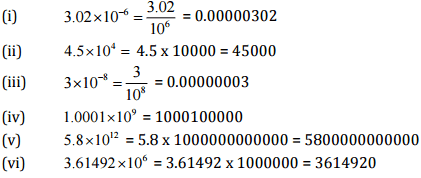Question 3:

Express the number appearing in the following statements in standard form: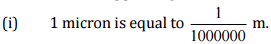(ii) Charge of an electron is 0.000,000,000,000,000,000,16 coulomb.

(iii) Size of a bacteria is 0.0000005 m.

(iv) Size of a plant cell is 0.00001275 m.

(v) Thickness if a thick paper is 0.07 mm.

(i) 1 micron = 1/1000000 = 1/106 = 1 x 10-6 m

[ii] Charge of an electron is 0.00000000000000000016 coulombs.

= 0.00000000000000000016 x 1019 / 1019  = 1.6x10-19 coulomb

[iii] Size of bacteria = 0.0000005 = 5/10000000 = 5x10-7

(iv) Size of a plant cell is 0.00001275 m = 0.00001275 x 105/105 = 1.245 x 10-5 m

(v) Thickness of a tliick paper = 0.07 mm = 7/100 mm = 7/102 = 7 x 10-2 mm

Question 4:

In a stack there are 5 books each of thickness 20 mm and 5 paper sheets each of thickness 0.016 mm. What is the total thickness of the stack?

Thickness of one book = 20 mm

Thickness of 5 books = 20 x 5 = 100 mm

Thickness of one paper = 0.016 mm

Thickness of 5 papers = 0.016 x 5 = 0.08 mm

Total thickness of a stack = 100 + 0.08 = 100.08 mm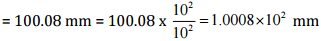Offer running on EduRev: Apply code STAYHOME200 to get INR 200 off on our premium plan EduRev Infinity!

,

,

,

,

,

,

,

,

,

,

,

,

,

,

,

,

,

,

,

,

,

,

,

,

,

,

,

;